# Scientific Notation Scientific Notation In scientific notation numbers

• Slides: 18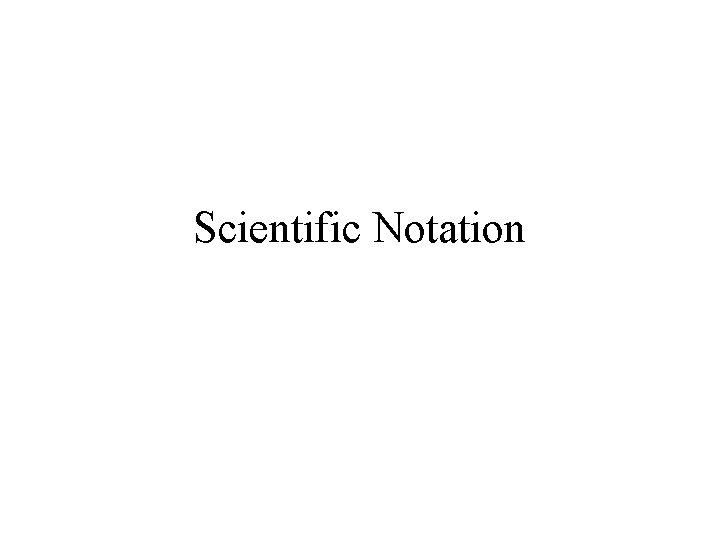Scientific NotationScientific Notation “In scientific notation, numbers are written in the form M x 10 n, where the factor M is a number greater than or equal to 1 but less than 10 and n is a whole number” (Modern Chemistry 50) Example: 2 x 102 m 1. 45 x 104 cm 8. 08 x 10 -3 mol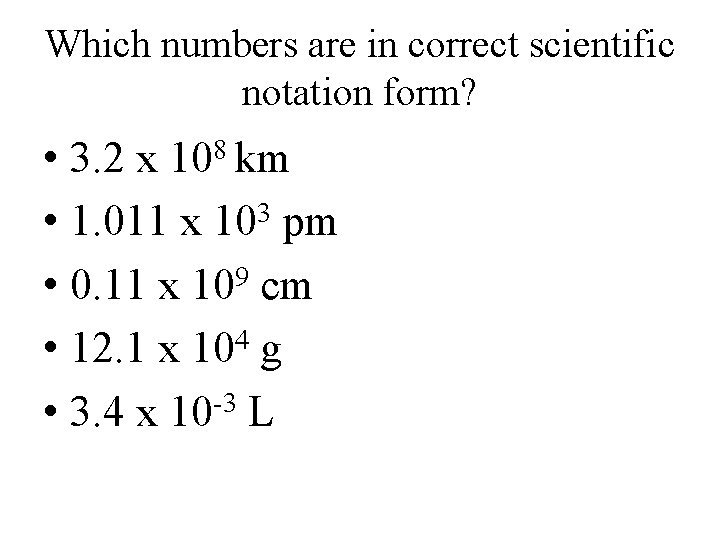Which numbers are in correct scientific notation form? • 3. 2 x • 1. 011 x 103 pm • 0. 11 x 109 cm • 12. 1 x 104 g • 3. 4 x 10 -3 L 8 10 kmScientific Notation “When numbers are written in scientific notation, only the significant figures are shown” (Teacher Edition 51)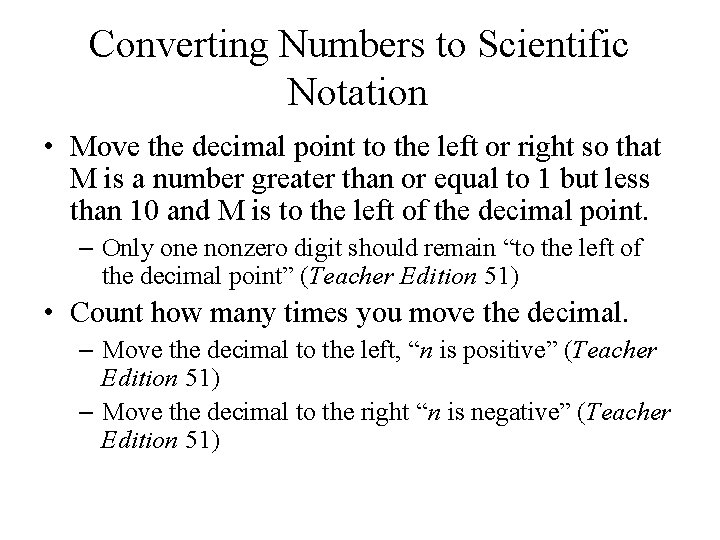Converting Numbers to Scientific Notation • Move the decimal point to the left or right so that M is a number greater than or equal to 1 but less than 10 and M is to the left of the decimal point. – Only one nonzero digit should remain “to the left of the decimal point” (Teacher Edition 51) • Count how many times you move the decimal. – Move the decimal to the left, “n is positive” (Teacher Edition 51) – Move the decimal to the right “n is negative” (Teacher Edition 51)Converting Numbers to Scientific Notation • Example: 234 000 m. L = 5420 g = 0. 00000024 m =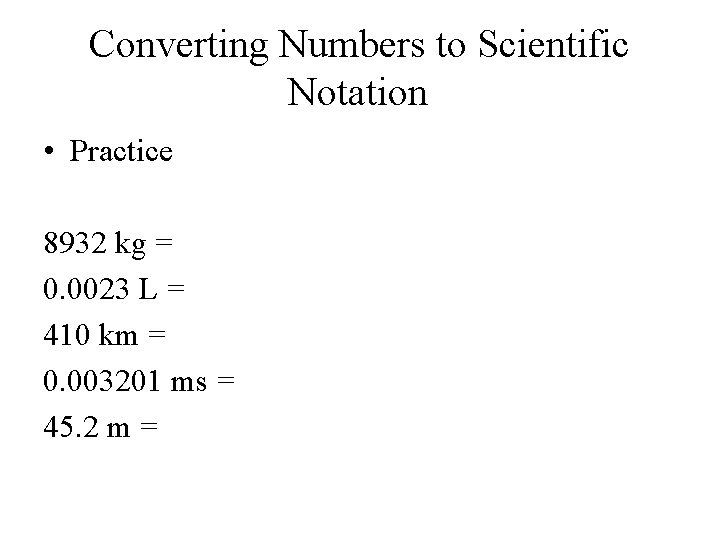Converting Numbers to Scientific Notation • Practice 8932 kg = 0. 0023 L = 410 km = 0. 003201 ms = 45. 2 m =Converting from Scientific Notation to Ordinary Notation • Look to the exponent –If the exponent is positive, move the decimal to the right n times –If the exponent is negative, move the decimal to the left n times M x 10 nConverting from Scientific Notation to Ordinary Notation • Example: 2. 3 x 105 m = 4. 5 x 10 -2 L =Converting from Scientific Notation to Decimal Form 3. 4 x 108 mm = 4. 56 x 102 kg = 1. 24 x 10 -7 s = 5. 41 x 10 -4 L = 4. 3 x 1012 g = 1. 3 x 10 -11 km =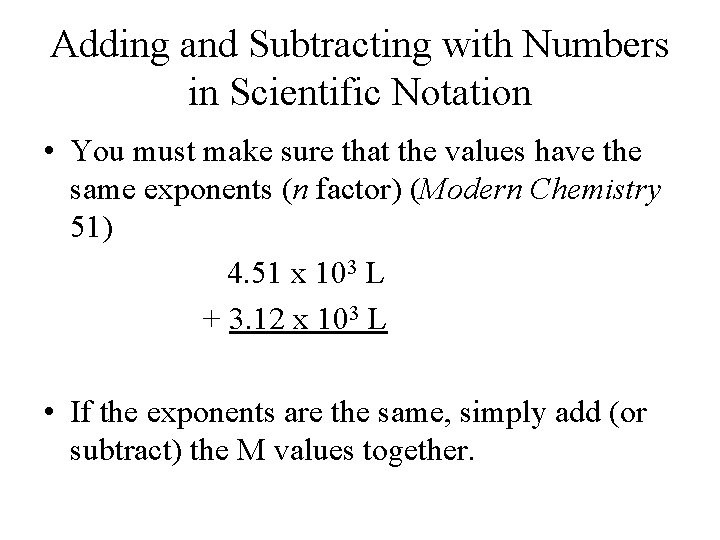Adding and Subtracting with Numbers in Scientific Notation • You must make sure that the values have the same exponents (n factor) (Modern Chemistry 51) 4. 51 x 103 L + 3. 12 x 103 L • If the exponents are the same, simply add (or subtract) the M values together.Adding and Subtracting in Scientific Notation • If the exponents are not the same, adjust one of the values so that the exponents match. 3. 41 x 105 m. L +2. 11 x 104 m. L Adjust either 3. 41 x 105 m. L by moving the decimal to the right one space or adjust 2. 11 x 104 m. L by moving the decimal to the left one space. 3. 41 x 105 m. L +. 211 x 105 m. L When adding and subtracting, the units will remain the same throughout the problem (Teacher Edition 51)Adding and Subtracting in Scientific Notation • Your answer may then need to be put in proper scientific notation. • Don’t forget to round to the proper number of decimal places according to the significant figure rules!Adding and Subtracting in Scientific Notation 4. 53 x 108 m. L +1. 3 x 107 m. L 5. 103 x 109 m - 3. 24 x 108 m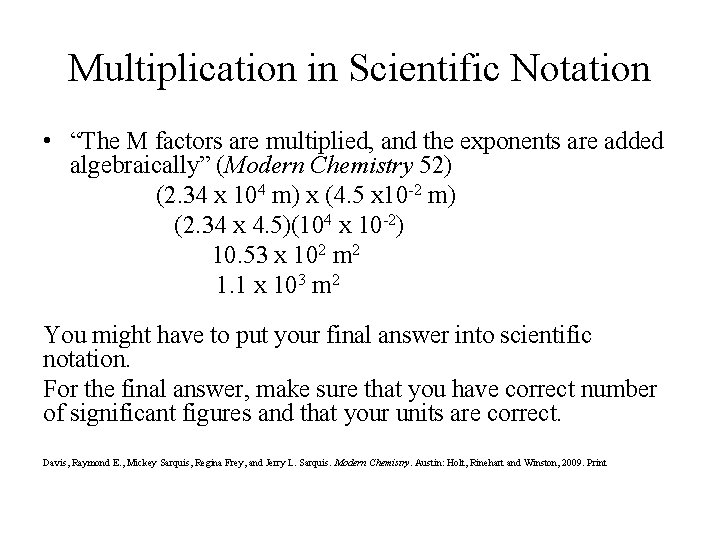Multiplication in Scientific Notation • “The M factors are multiplied, and the exponents are added algebraically” (Modern Chemistry 52) (2. 34 x 104 m) x (4. 5 x 10 -2 m) (2. 34 x 4. 5)(104 x 10 -2) 10. 53 x 102 m 2 1. 1 x 103 m 2 You might have to put your final answer into scientific notation. For the final answer, make sure that you have correct number of significant figures and that your units are correct. Davis, Raymond E. , Mickey Sarquis, Regina Frey, and Jerry L. Sarquis. Modern Chemistry. Austin: Holt, Rinehart and Winston, 2009. PrintDivision in Scientific Notation • “The M factors are divided, and the exponent of the denominator is subtracted from that of the numerator” (Modern Chemistry 52) 4. 311 x 104 g = 2. 12 x 102 mol You might have to put your final answer into scientific notation. For the final answer, make sure that you have correct number of significant figures and that your units are correct.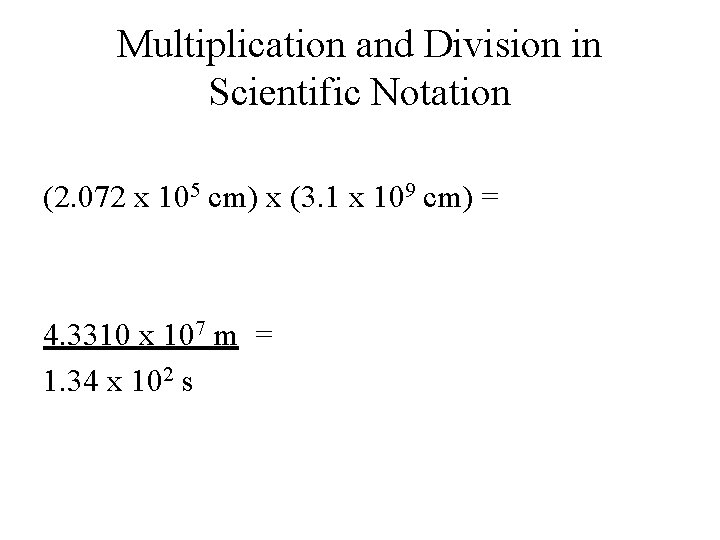Multiplication and Division in Scientific Notation (2. 072 x 105 cm) x (3. 1 x 109 cm) = 4. 3310 x 107 m = 1. 34 x 102 sWorks Cited Davis, Raymond E. , Mickey Sarquis, Regina Frey, and Jerry L. Sarquis. Modern Chemistry. Austin: Holt, Rinehart and Winston, 2009. Print. Davis, Raymond E. , Mickey Sarquis, Regina Frey, and Jerry L. Sarquis. Modern Chemistry Teacher Edition. Austin: Holt, Rinehart and Winston, 2006. Print.